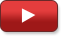Explore BrainMass

# An Introduction to Complex Numbers

In this lecture, Academic Expert John Gillis introduces the mathematical concept of complex numbers. John begins with a section illustrating and describing the set of complex numbers. His second section is about how to perform operations (addition, subtraction, multiplication, and division) on the complex numbers. Also covered is the imaginary unit i, the Complex Plane, and the FOIL method.

In this series: 3 Videos | 00:13:08 Total Length

The set of complex numbers is identified; the imaginary unit i is also included and the viewer is introduced to the complex plane.

Part #: 1 | 03:46This video covers how to add, subtract, multiply, and divide complex numbers, with several examples included.

Part #: 2 | 06:20
\$2.49More examples are included, and the video wraps up with a practice test.

Part #: 3 | 03:02
\$2.49